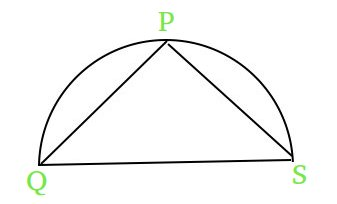Open in App
Not now

# Maximize a value for a semicircle of given radius

• Difficulty Level : Medium
• Last Updated : 07 Jul, 2022

We are given a semi circle with radius R. We can take any point on the circumference let it be P.Now, from that point P draw two lines to the two sides of diameter. Let the lines be PQ and PS.
The task is to find the maximum value of expression PS2 + PQ for a given R.Examples :

```Input : R = 1
Output : 4.25
(4*1^2 + 0.25) = 4.25

Input : R = 2
Output : 16.25
(4 * 2^2 + 0.25)= 16.25```

Let F = PS^2 + PQ. We know QS = 2R (Diameter of the semicircle)
-> We also know that triangle PQS will always be right angled triangle irrespective of the position of point P on the circumference of circle

```1.)QS^2 = PQ^2 + PS^2 (Pythagorean Theorem)

2.) Adding and Subtracting PQ on the RHS
QS^2 = PQ^2 + PS^2 + PQ - PQ

3.) Since QS = 2R
4*R^2 + PQ - PQ^2 = PS^2 + PQ
=> 4*R^2 + PQ - PQ^2 = F

4.) Using the concept of maxima and minima
differentiating F with respect to PQ and equating
it to 0 to get the point of maxima for F i.e.
1 - 2 * PQ = 0
=> PQ = 0.5

5.) Now F will be maximum at F = 4*R^2 + 0.25 ```

## C++

 `// C++ program to find``// the maximum value of F``#include ``using` `namespace` `std;` `// Function to find the``// maximum value of F``double` `maximumValueOfF (``int` `R)``{``    ``// using the formula derived for``    ``// getting the maximum value of F``    ``return` `4 * R * R + 0.25;    ``}``    ` `// Drivers code``int` `main()``{``    ``int` `R = 3;``    ``printf``(``"%.2f"``, maximumValueOfF(R));``    ``return` `0;``}`

## JAVA

 `// JAVA program to find``// the maximum value of F``import` `java.io.*;` `class` `GFG``{``    ` `    ``// Function to find the``    ``// maximum value of F``    ``static` `double` `maximumValueOfF (``int` `R)``    ``{``        ` `        ``// using the formula derived for``        ``// getting the maximum value of F``        ``return` `4` `* R * R + ``0.25``;    ``    ``}``    ` `    ``// Driver code``    ``public` `static` `void` `main (String[] args)``    ``{``        ``int` `R = ``3``;``        ``System.out.println(maximumValueOfF(R));``    ``}``        ` `}` `// This code is contributed``// by anuj_67.`

## Python3

 `# python program to find``# the maximum value of F` `# Function to find the``# maximum value of F``def` `maximumValueOfF (R):``    ` `    ``# using the formula derived for``    ``# getting the maximum value of F``    ``return` `4` `*` `R ``*` `R ``+` `0.25` `     ` `# Drivers code``R ``=` `3``print``(maximumValueOfF(R))` `# This code is contributed by Sam007.`

## C#

 `// C# program to find the``// maximum value of F``using` `System;` `class` `GFG``{``    ` `    ``// Function to find the``    ``// maximum value of F``    ``static` `double` `maximumValueOfF (``int` `R)``    ``{``        ` `        ``// using the formula derived for``        ``// getting the maximum value of F``        ``return` `4 * R * R + 0.25;    ``    ``}``    ` `    ``// Driver code``    ``public` `static` `void` `Main ()``    ``{``        ``int` `R = 3;``        ``Console.WriteLine(maximumValueOfF(R));``    ``}``        ` `}` `// This code is contributed by Sam007.`

## PHP

 ``

## Javascript

 ``

Output :

`36.25`

Time Complexity: O(1)
Auxiliary Space: O(1)

My Personal Notes arrow_drop_up